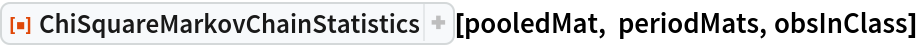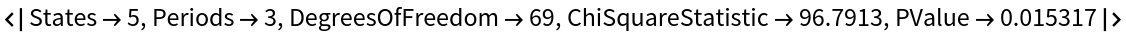Function Repository Resource:

# ChiSquareMarkovChainStatistics

Calculate a chi-square statistic reflecting the homogeneity of a Markov chain's transition matrix over several time periods

Contributed by: Konstantin Nosov
 ResourceFunction["ChiSquareMarkovChainStatistics"][poolmat,permats,classcts] tests the homogeneity of a Markov chain's transition matrix poolmat across all time periods, where permats is the list of transition matrices for each period and classcts is the number of observations.

## Details

The entire sample (a sequence of states of a Markov chain) is assumed to be divided into T periods, where T is Length[permats].
If the Markov chain has N states, the pooled matrix poolmat is an N×N matrix.
If the number of periods is T, permats is a T×N×N list of period matrices, where permats[[t]] is the transition matrix for the tth period.
The number of observations in class, classcts, is a T×N list of lists where classcts[[t,i]] denotes the number of observations initially falling into the ith class (state) within the tth sub-period.
ResourceFunction["ChiSquareMarkovChainStatistics"] returns an Association with the following keys:
 "States" number of states "Periods" number of periods "DegreesOfFreedom" degrees of freedom "ChiSquareStatistics" χ2 statistics "PValue" p-value

## Examples

### Basic Examples (1)

Return χ2 statistic and associated values for a Markov chain with 5 states over 3 time periods:In:=Out=Konstantin

## Version History

• 1.0.0 – 18 May 2021